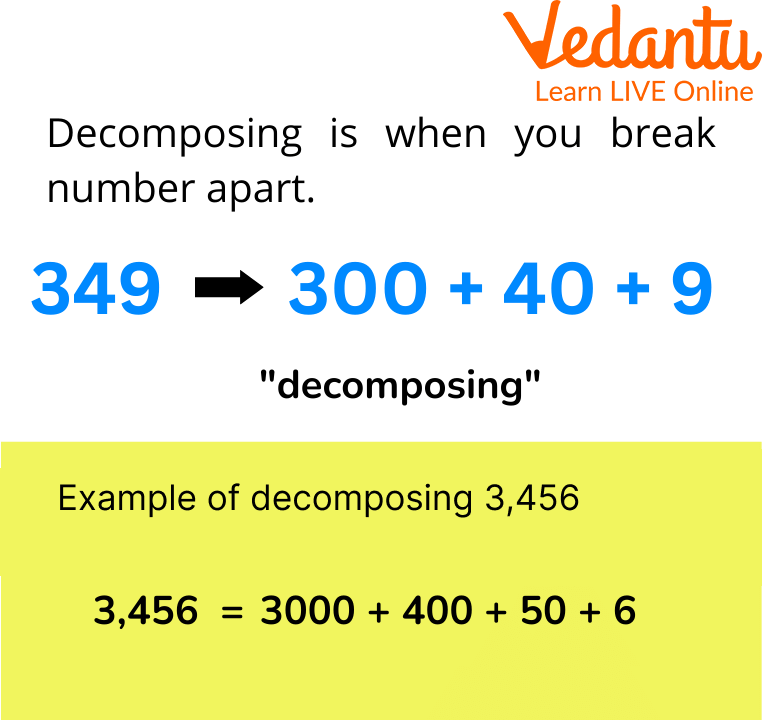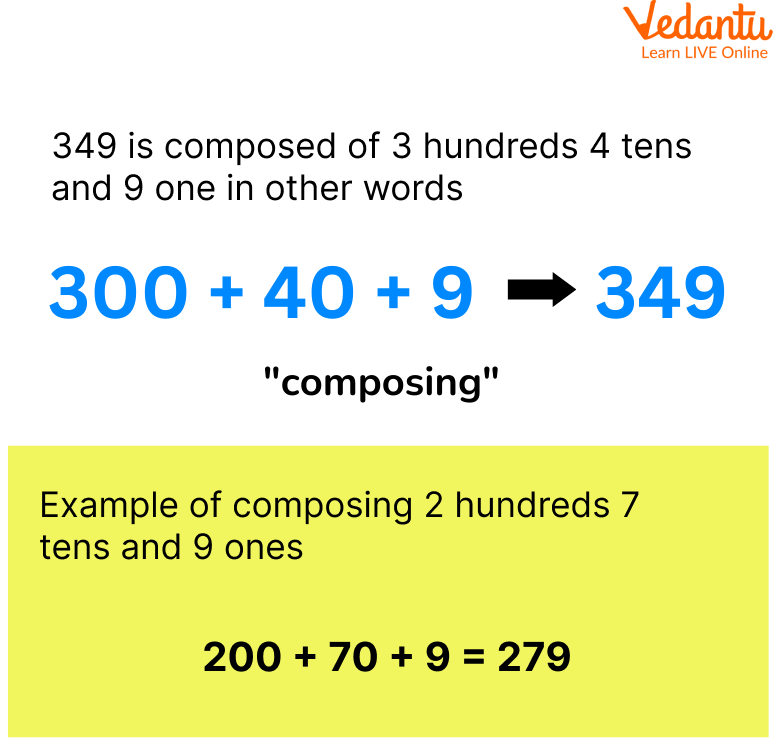Courses
Courses for Kids
Free study material
Free LIVE classes
More

# Composing and Decomposing NumbersLIVE
Join Vedantu’s FREE Mastercalss

## A Brief Introduction about Composing and Decomposing Numbers

Do you know what composing and decomposing numbers mean? In this article, you will gain complete information about composing and decomposing numbers for kindergarten, along with the difference between them. This article will also assist teachers and parents in gaining new ideas about how to teach composing and decomposing numbers to children. Some composing and decomposing numbers worksheets are given to help students in mastering the concepts. So let's begin with the topic.

## Define Composing and Decomposing Numbers for Kindergarten

Composing in maths is defined as a way of putting numbers together using their parts. Here many values are added together to obtain the total value. A number is represented as the sum of its various place values. When such values are combined or put together, the result is a standard form number. For example, 349 = 300 + 40 + 9.Composing Numbers

Decomposing in maths is defined as a way of breaking down numbers into parts. A number can be broken down into its various parts based on place values. For example, 3,456 = 3000 + 400 + 50 + 6Decomposing Numbers

## What is the Difference Between Composing and Decomposing Numbers?

We have learned about composing and decomposing numbers for kindergarten. Now, what is the difference between composing and decomposing numbers? Some differences between composing and decomposing numbers are stated below:

 S. No. Composing Numbers Decomposing Numbers 1 It helps the students understand how individual components fit together to form a whole. It helps the students in understanding how the whole is broken down into individual components. 2 For example: composing 20 and 3 gives 23. For example, decomposing 16 gives 10 and 6. 3 Composing numbers yields a unique result. A number can be decomposed in more than two ways. 4 Composing various numbers gives the standard form of the number. Decomposing of numbers breaks the standard form into various numbers.

## How to Teach Composing and Decomposing Numbers

Let us now discuss some steps on how to teach composing and decomposing numbers.

• Using flashcards and anchor chart:

Flashcards and anchor chart made with the students helps them in learning the concept of composing and decomposing numbers

• Introducing whole and parts:

Whole groups and parts help the students in learning how to compose and decompose the numbers easily

• Using maths games:

Learning to compose and decompose numbers using games is very beneficial to build one's problem-solving skills. These can also be board games.

## Solved Examples

Q1. Compose the number 9000+20+3 and verify the result.

Ans: To compose the given number, write it in terms of its place value and then perform addition corresponding to each place value, i.e. 9000+23, which equals the number 9023.

To verify the result, we need to decompose the obtained number, which is done by breaking the number in terms of its corresponding place value, i.e. thousands, hundreds, tens, and ones. 9000+000+20+3, which equals 9000+20+3. Thus the answer is verified.

Hence the composed number is 9023.

Q2. Decompose the number 386.

Ans: To decompose the number, we need to break the given number, 386, in terms of its corresponding place value. As the number has hundreds, tens and ones place values, it can be broken down as 300+80+6.

Hence the result of the decomposing number 386 is 300+80+6.

## Practice Questions

Q1. Compose the given number

10,000 + 4,000 + 20 + 9.

Ans: 14029

Q2. Decompose the number 56.

Ans: 50 + 6

Q3. Compose the given number and verify the result obtained.

100 + 30 + 2

Ans: 132

## Composing and Decomposing Numbers Worksheet

Q1. Decompose the given number 4156.

Ans: 4000+100+50+6

Q2. Compose the number, 5000 + 20 + 8.

Ans: 5028

Q3. Decompose the given number 963.

Ans: 900+60+3

## Summary

Summing up here with composing and decomposing numbers for kindergarten. In this article, we studied composing which means putting numbers together, and decomposing which means breaking down the numbers in parts. Here we have covered; what is the difference between composing and decomposing numbers along with the methods of how to teach composing and decomposing numbers. Some practice questions are given based on the solved examples that will help the students grab the topic easily. Composing and decomposing numbers worksheets are also provided for practice.

Last updated date: 27th Sep 2023
Total views: 91.2k
Views today: 2.91k

## FAQs on Composing and Decomposing Numbers

Q1. Are ten frames useful in learning to decompose numbers?

Yes, the ten frames are useful in learning decomposing numbers because the numbers are arranged in two rows of five each. So it becomes easy for students to understand how the numbers are broken down into parts.

Q2. What are the benefits of 2nd-grade decomposing numbers worksheets

Decomposing numbers 2nd-grade worksheets will help kids understand how bigger numbers are formed. This will help them retain the concepts of place value as well as what position multiple digits hold.

Q3. Why is learning to decompose and compose numbers important?

The learning of composing and decomposing numbers will help the students become more fluent in their calculations. It also helps to build a number sense. Students will be able to relate to maths when they learn about breaking down and reassembling.